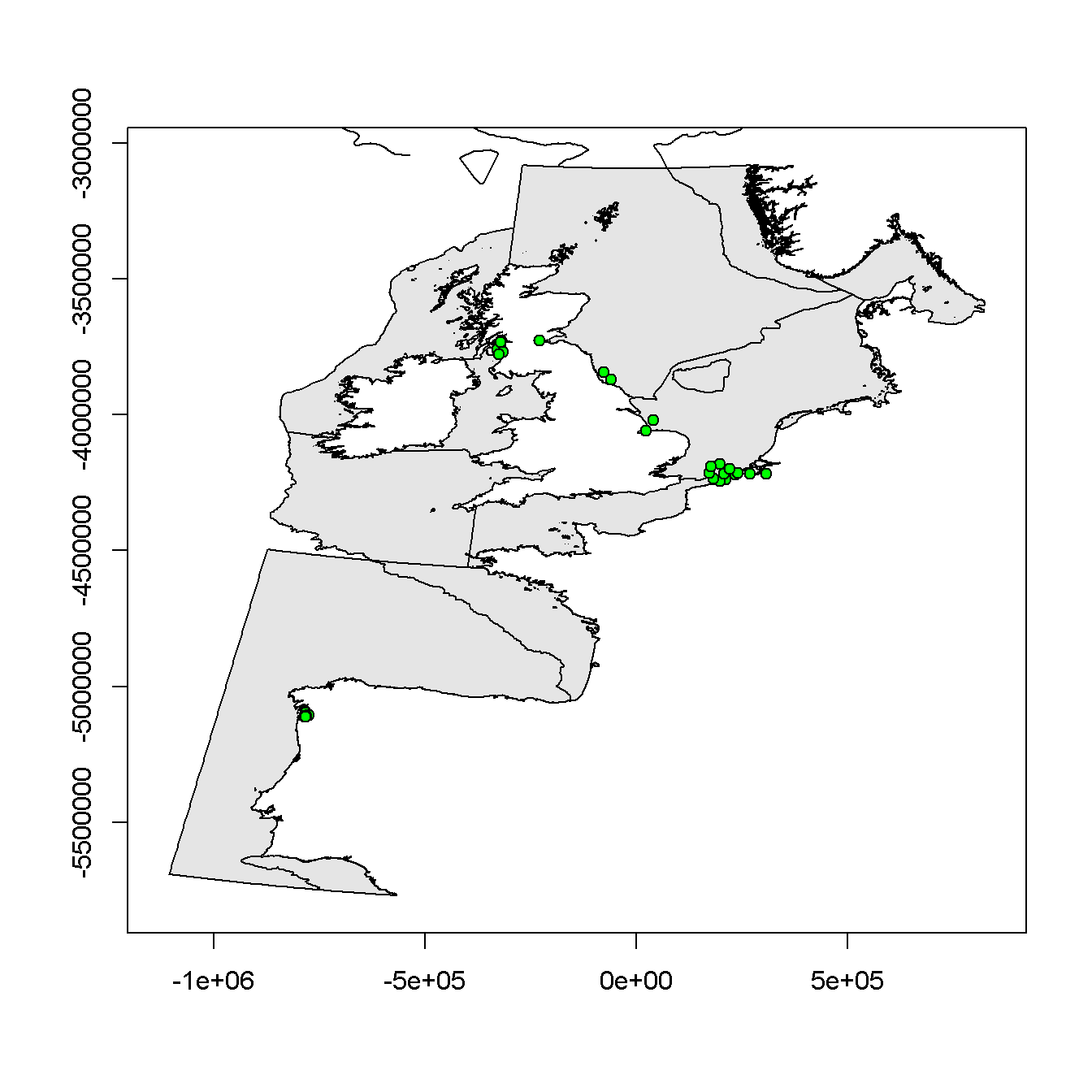## Assessment Criteria for Polybrominated Diphenyl Ethers

This document summarises the discussions at OSPAR MIME 2018 on setting Background Assessment Concentrations (BACs) for Polybrominated Diphenyl Ethers (PBDEs). It builds on discussions at MIME 2017.

The first section describes the methodology for estimating BACs. The next section then develops BACs for PBDEs in sediment, shellfish and fish.

### BAC estimation methodology

BACs are used to test whether concentrations are at background levels for naturally occurring substances, or ‘close to background’ for synthetics. This is done by testing the null hypothesis $${\text{H}_0: \mu \geq \text{BAC}}$$ against the alternative hypothesis $${\text{H}_1: \mu < \text{BAC}}$$, where $$\mu$$ is the mean concentration (in the final monitoring year). The test is precautionary since concentrations are considered to be above background ($${\text{H}_0}$$) unless there is sufficient evidence to show otherwise ($${\text{H}_1}$$).

The BAC is chosen to give a 90% power of rejecting $$\text{H}_0$$ when $$\mu = \text{BC}$$, where BC is the Background Concentration. The BC of natural occurring substances is estimated using data from near-pristine locations (assuming they exist) or, for sediment concentrations, from cores. The BC of synthetic substances is zero, and to construct the BAC it is necessary to replace the BC by a Low Concentration (LC) which is typically taken to be twice the Quasimeme standard error. For the purposes of this section, the BC (or LC) is assumed known.

Power depends on the statistical test used, the number of years of data, and the variability in the data. It is necessary to standardise these to construct a BAC that can applied across the OSPAR area. Specifically, it is assumed that:

• there is a 10-year monitoring programme with the same sampling protocol followed every year (or equivalently and more realistically, a 20-year monitoring programme with sampling every two years)
• the test is a one-tailed t-test at the 5% significance level based on the temporal trend regression model fitted to the monitoring data
• the variability in the data is typical of that found in MIME monitoring data; this is developed later, but for now it is assumed that the variability is characterised by a parameter $$\psi$$

Assume that the concentration measurements each year are summarised by an annual index on the logarithmic scale (e.g. the median log concentration or the mean log concentration). Let $$\mathbf y$$ be the vector (length 10) of annual contaminant indices and assume that

$$\quad \mathbf y = \mathbf f + \mathbf \epsilon$$

where $$\mathbf f$$ represents a smooth systematic trend in contaminant levels over time and $$\mathbf \epsilon$$ represents random variation, assumed to be independent between years and normally distributed with zero mean and standard deviation $$\psi$$. Let $$\mathbf{X}$$ be the design matrix used to estimate the systematic trend $$\mathbf f$$. The choice of $$\mathbf{X}$$ is also discussed later, but it could correspond to a linear trend or a smooth on a fixed number of degrees of freedom. It is assumed that $$\mathbf{X}$$ estimates $$\mathbf f$$ with no bias. Let $$r$$ be the rank of $$\mathbf X$$ and $$\nu = 10 - r$$ be the residual degrees of freedom associated with $$\mathbf X$$. Let

$$\quad \mathbf{H = X(X'X)^{-1}X'}$$

be the hat matrix. The fitted trend is then $$\mathbf {\hat{f} = Hy}$$, which has variance $$\mathbf H \psi^2$$.

A one-tailed t-test at the 5% significance level is used to test $${\text{H}_0}$$ vs $${\text{H}_1}$$. Let

$$\quad T = \frac {\hat {\mathbf f} _{10} \text{ }- \text{log BAC}} {\hat \psi \sqrt {\mathbf H_{10,10}} }$$

where the 10 suffix picks out the fitted value and its variance in the final monitoring year, and where $$\hat {\psi^2}$$ is the usual unbiased estimator of $$\psi^2$$. Then $${\text{H}_0}$$ is rejected in favour of $${\text{H}_1}$$ if $$T < t_{\text{crit}}$$, where $$t_{\text{crit}}$$ is the 0.05 quantile of a central t-distribution on $$\nu$$ degrees of freedom, denoted $$(t ; \nu).$$ That is, the value such that

$$\quad \text{Prob} \big( (t ; \nu) < t_{\text{crit}} \big) = 0.05$$

When $$\mathbf f_{10} = \text{log BAC}$$, $$T$$ has a central t-distribution $$T \sim (t; \nu)$$. More generally, T has a non-central t-distribution $$T \sim (t; \nu; \delta)$$ on $$\nu$$ degrees of freedom and non-centrality parameter

$$\quad \delta = \frac {\mathbf f_{10} - \text{log BAC}} {\psi \sqrt {\mathbf H_{10,10}} }$$

The BAC is chosen to give 90% power when $$\mathbf f_{10} = \text {log BC}$$. Let $$\delta _ \text {crit}$$ be the value that satisfies

$$\quad \text{Prob} \big( (t ; \nu; \delta _ \text{crit} ) < t_{\text{crit}} \big) = 0.90$$

Then the BAC is given by

$$\quad \text {log BAC} = \text {log BC} - \delta _ \text {crit} \psi \sqrt {\mathbf H_{10,10}}$$

or equivalently

$$\quad \text {BAC} = \text {BC} \text {exp} \big( - \delta _ \text {crit} \psi \sqrt {\mathbf H_{10,10}} \big)$$

Two problems remain: choosing the appropriate form of $$\mathbf X$$ and hence $$\mathbf H$$ and estimating a suitable value of $$\psi$$. The methods for doing both have been updated since the last time BACs were constructed (around 2005 for compounds in other determinand groups) to accommodate developments in the trend assessment methodology. A summary of the changes can be found in the discussions at MIME 2017.

First consider the choice of $$\mathbf X$$. A time series with 10 years of data is assessed by fitting a linear trend and smooths on 2 and 3 degrees of freedom (df) and choosing the optimal model based on AICc. In practice, most time series reduce to a linear trend. However, the smooth on 2 df gives BACs that are closest to the previous approach and hence are used here. The corresponding values of $$\delta _ \text{crit}$$ and $$\mathbf H$$ leads to BACs of the form:

$$\quad \text {BAC} = \text {BC} \text {exp} (2.51 \psi )$$

Now consider the choice of $$\psi$$. The concentration measurements are modelled in a mixed modelling framework. This gives estimates of the between-sample and between-year random variation for each time series, denoted here as $$\sigma_{i,\text {sample}}^2$$ and $$\sigma_{i,\text {year}}^2$$ with the $$i$$ used to index the time series. Analytical variability and the number of samples per year have tended to evolve in most time series (getting lower and fewer respectively) so, to characterise recent performance, the analytical variability $$\sigma_{i,\text {analytical}}^2$$ for time series was based on the median reported uncertainty in the last three monitoring years. Similarly, the number of samples per year $$n_i$$ for time series $$i$$ was taken to be the median number of samples in the last three monitoring years. The residual variance for time series $$i$$ was then estimated to be

$$\quad \psi _i ^2 = \sigma _ {i, \text {year}} ^2 + \frac 1 {n_i} \big (\sigma _ {i, \text {sample}} ^2 + \sigma _ {i, \text {analytical}} ^2 \big )$$

The value of $$\psi$$ typical of MIME monitoring data was then estimated by fitting a robust loess smoother to the $$\text {log} \psi _ i$$ as a function of the fitted log mean concentration in the final monitoring year, with a span of 1 and weighted by the number of years in each time series. The (back-transformed) fitted value of the loess smoother at the BC (LC) is taken to be the typical $$\psi$$ at background (low) concentrations. When the BC (LC) is outside the range of the data, the fitted value at the closest observed mean concentration is used.

One final important caveat. BACs are statistical constructs and there is no guarantee that they are environmentally relevant. They should be rejected if they are higher than the EAC or equivalent (if it exists) and if they are too high based on expert judgement. For PBDES, the Canadian Federal Environmental Quality Guidelines (FEQGs) are used as EAC equivalents.

### BAC estimates

MIME agreed to trial BACs of:

• sediment: 0.05 $$\mu$$g/kg dw for all PBDE compounds (normalised to 2.5% organic carbon except for the Iberian Sea and the Gulf of Cadiz)
• shellfish: 0.011 $$\mu$$g/kg ww for BDE47
• fish: 0.012 $$\mu$$g/kg ww for all PBDE compounds

Further work is required to develop BACs for PBDEs in shellfish, probably involving some analysis of passive sampling data.

The tabs below describe how these conclusions were reached.

#### Sediment

The Quasimeme constant error is 0.05 $$\mu$$g/kg dw for all determinands, so the standard choice of LC would be 0.1 $$\mu$$g/kg dw. However, this was considered too high because many time series had mean concentrations around 0.1 $$\mu$$g/kg dw even in areas that would not be considered background. Instead, an LC of 0.01 $$\mu$$g/kg dw was used, a value that was typical of the lowest mean concentrations observed for most PBDE compounds (see first tab below). (Note that this was also the approach adopted in discussions at MIME 2017, although the text does not make this clear.)

The first tab below shows, by determinand, the estimates of $$\psi$$ from each time series plotted against the estimated mean concentration in the final monitoring year. Mean concentrations in the final monitoring year are normalised to 2.5% organic carbon for Belgium, the Netherlands, Sweden and the United Kingdom but are not normalised for Spain. The points, coloured by contracting party, reveal systematic differences in variability between contracting parties which could be due to differences in the number of samples taken, analytical and reporting quality control, or natural variability in concentrations due to choice of monitoring stations or other environmental drivers. The fitted loess smoother (black line) with 95% pointwise confidence limits (dashed lines) is also shown. The three vertical lines are the LC (grey), the BAC (blue) constructed using the methodology described earlier and the FEQG (green). Note that there were too few data to estimate a BAC for BDE85 and the FEQG for BD183 is not plotted because it is off the scale of the plot.

The second tab gives the estimates of $$\psi$$ typical of MIME monitoring data at concentrations close to the LC, with lower and upper 95% confidence limits, and the BACs derived from them. The BACs are expressed as $$\mu$$g/kg dw normalised to 2.5% organic carbon for normalised sediments and $$\mu$$g/kg dw for non-normalised sediments.

The BACs are usually well below the FEQGs, although they are relatively close for BDE99 and BD100, so in this sense they are environmentally acceptable. However, the wide variation in BACs between compounds (0.02 - 0.19 $$\mu$$g/kg dw) was considered unsatisfactory, as it tended to reflect variation in analytical performance rather than environnmental characteristics, and as it often resulted in higher BACs for compounds that would be expected to have lower concentrations based on a typical PBDE profile. It was therefore decided to use the BAC for BDE47 of 0.05 $$\mu$$g/kg dw for all compounds. The value for BDE47 was chosen because a) it is based on the most data; b) BDE47 has one of the lower values of $$\psi$$ and hence one of the lower BACs; c) the BAC is below the mean concentration observed in most BDE47 time series, particularly in areas that would not be considered background.

The remaining tabs show status plots for each PBDE using a BAC of 0.05 $$\mu$$g/kg dw. Points are coloured

• blue if the mean concentration is significantly (p < 0.05) below the BAC
• green if the mean concentration is significantly (p < 0.05) below the FEQG
• red if the mean concentration is not significantly below the FEQG

##### Variability by country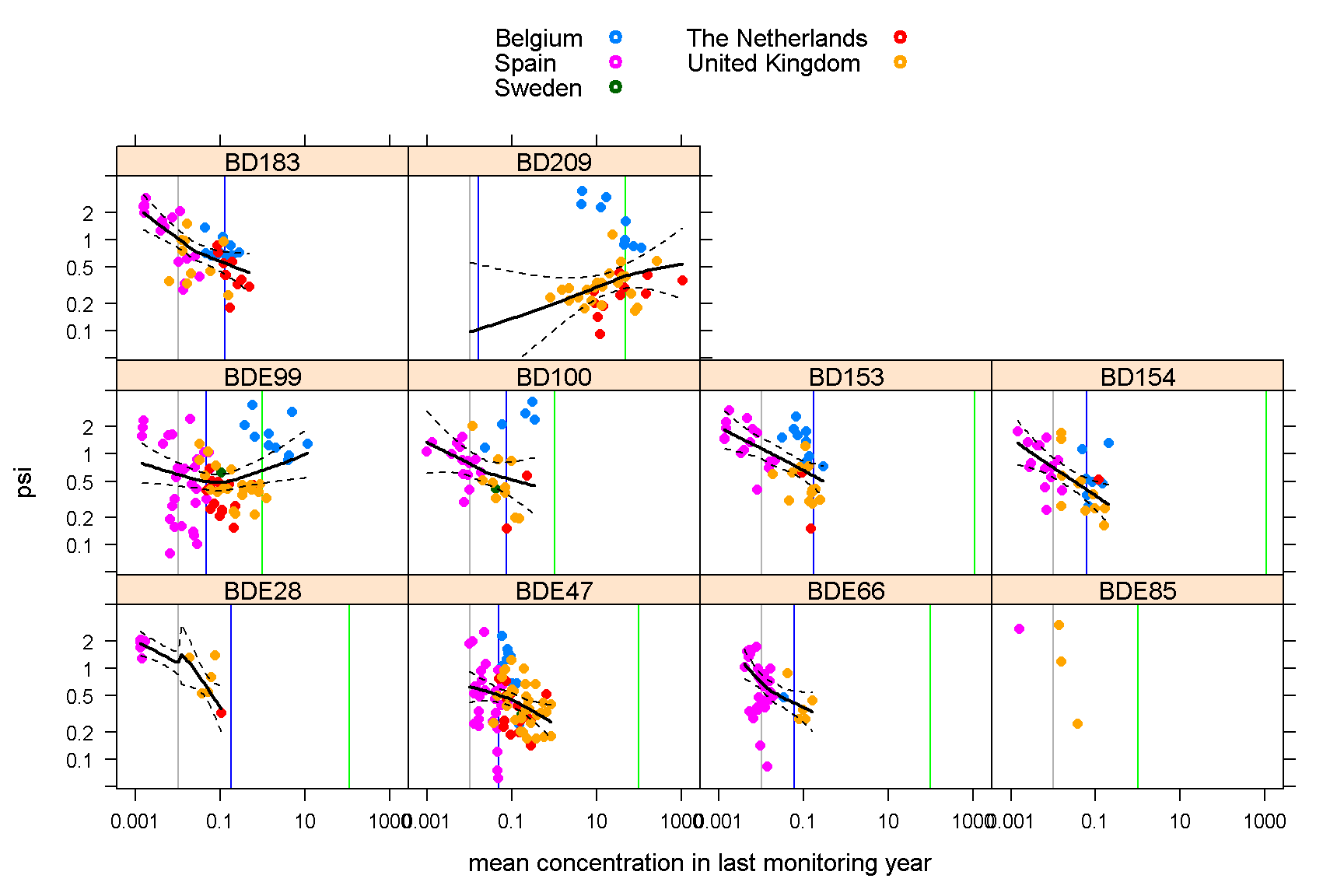##### BACs
LC psi lower upper BAC FEQG
BDE28 0.01 1.18 0.85 1.63 0.19 110.0
BDE47 0.01 0.62 0.42 0.92 0.05 97.5
BDE66 0.01 0.70 0.57 0.86 0.06 97.5
BDE85 0.01 1.0
BDE99 0.01 0.60 0.45 0.80 0.04 1.0
BD100 0.01 0.79 0.57 1.08 0.07 1.0
BD153 0.01 1.15 0.88 1.50 0.18 1100.0
BD154 0.01 0.71 0.55 0.91 0.06 1100.0
BD183 0.01 1.03 0.82 1.30 0.13 14000.0
BD209 0.01 0.19 0.09 0.39 0.02 47.5

##### BDE28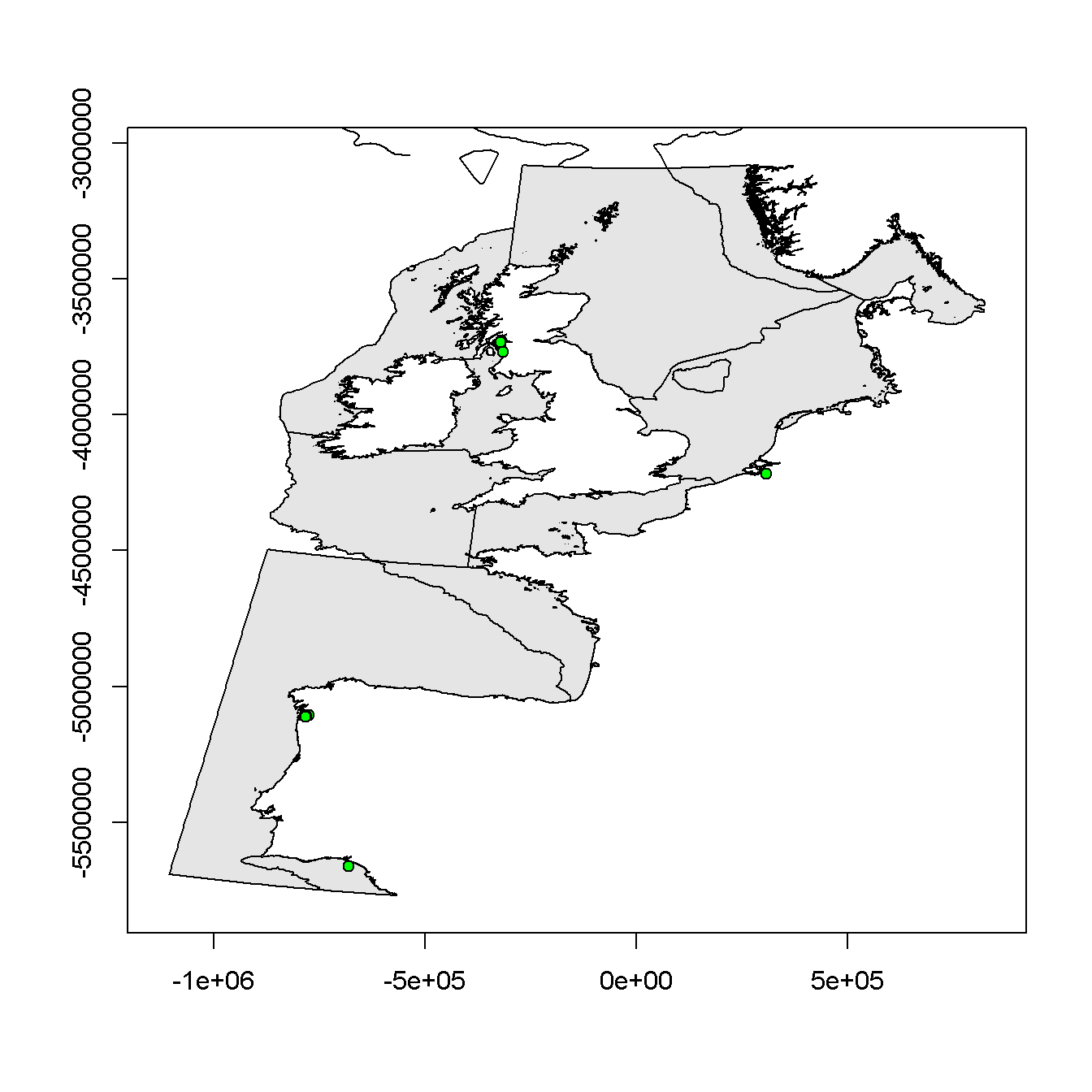##### BDE47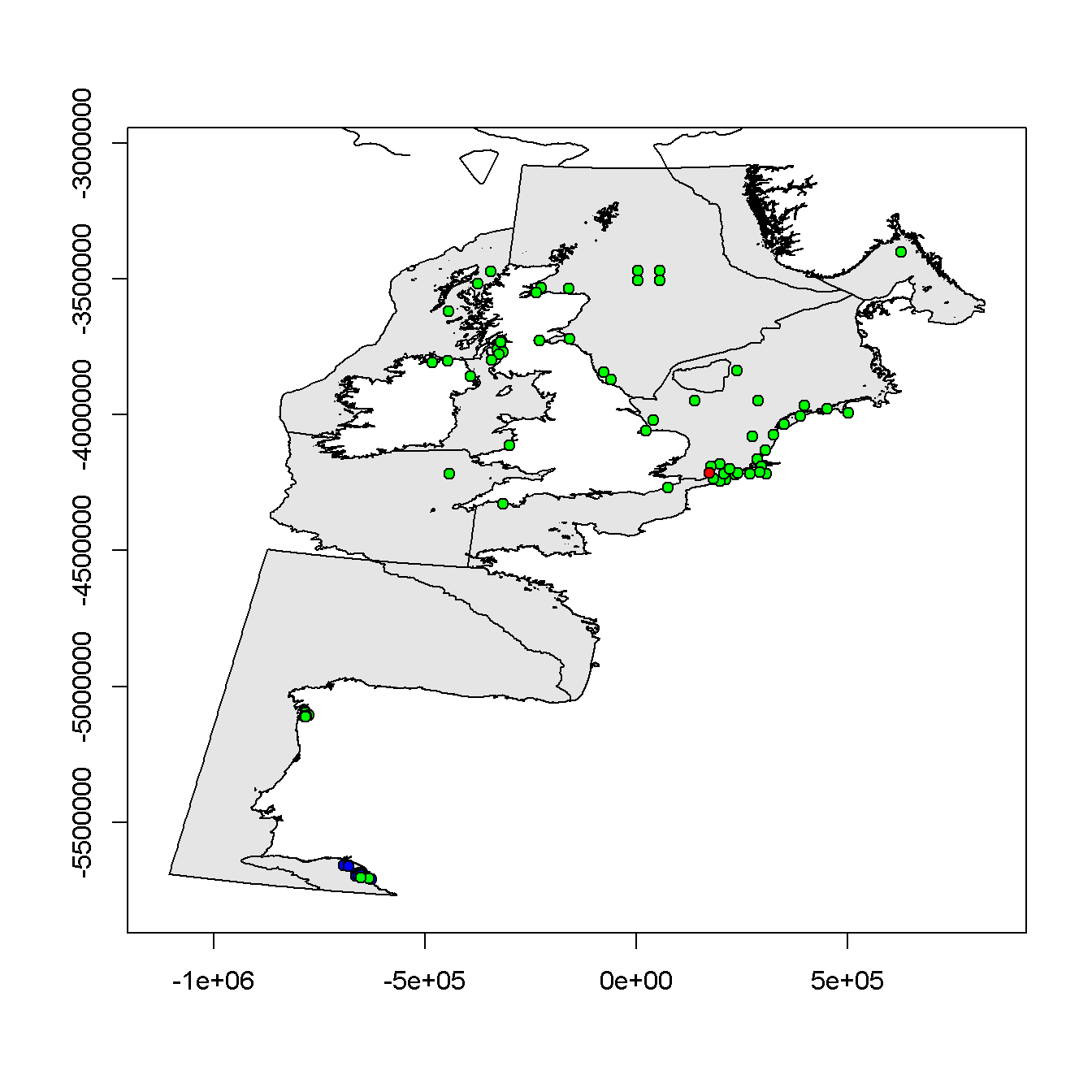##### BDE66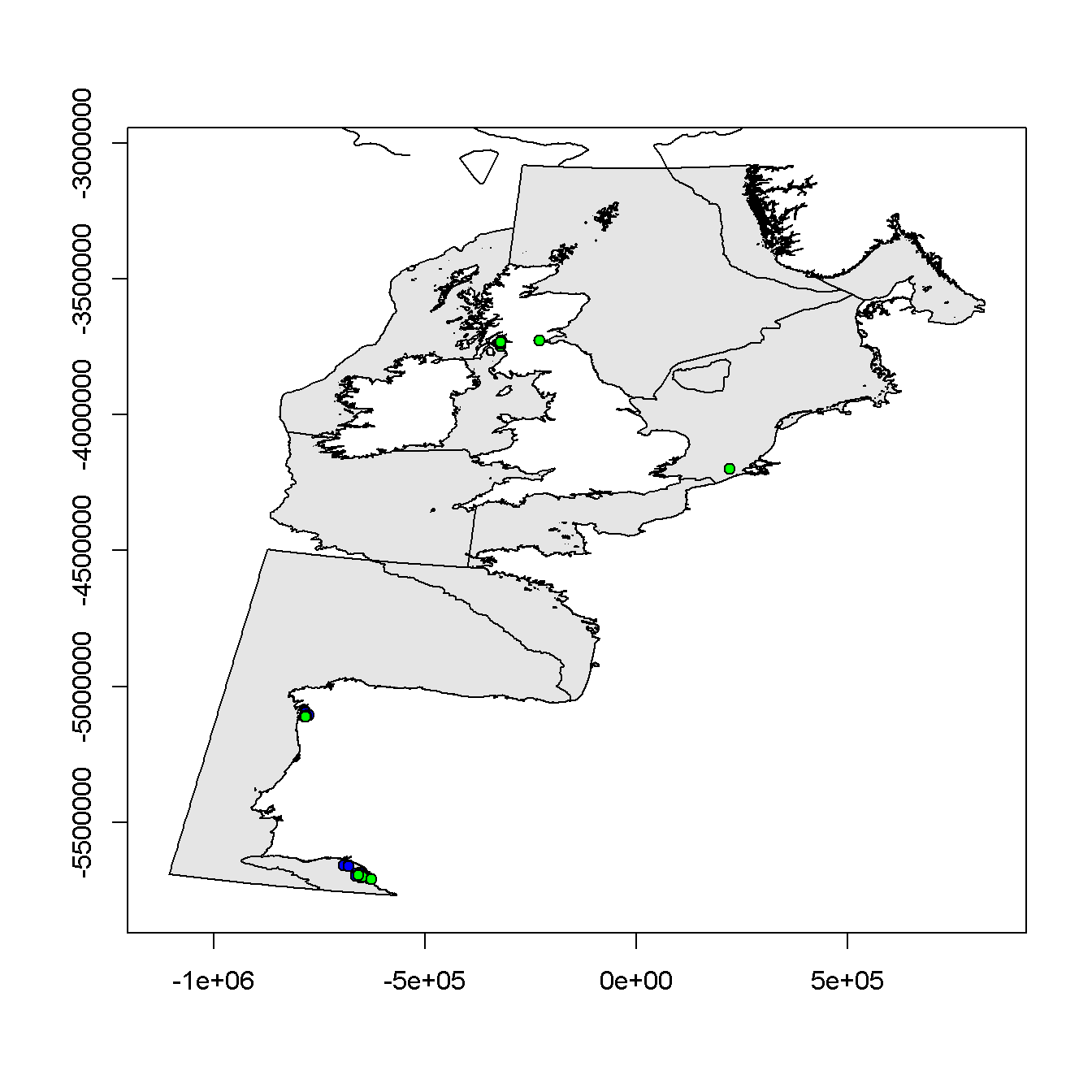##### BDE85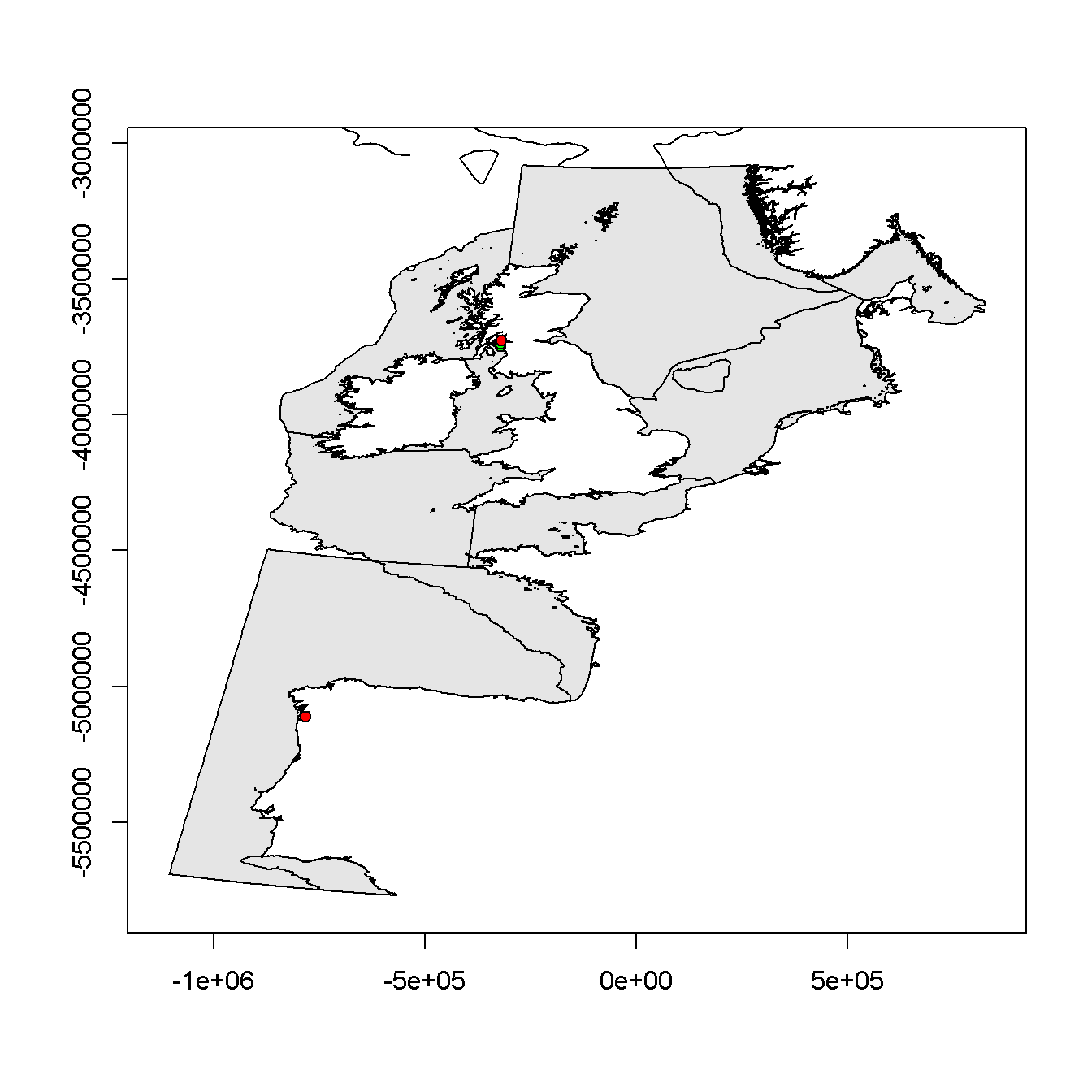##### BDE99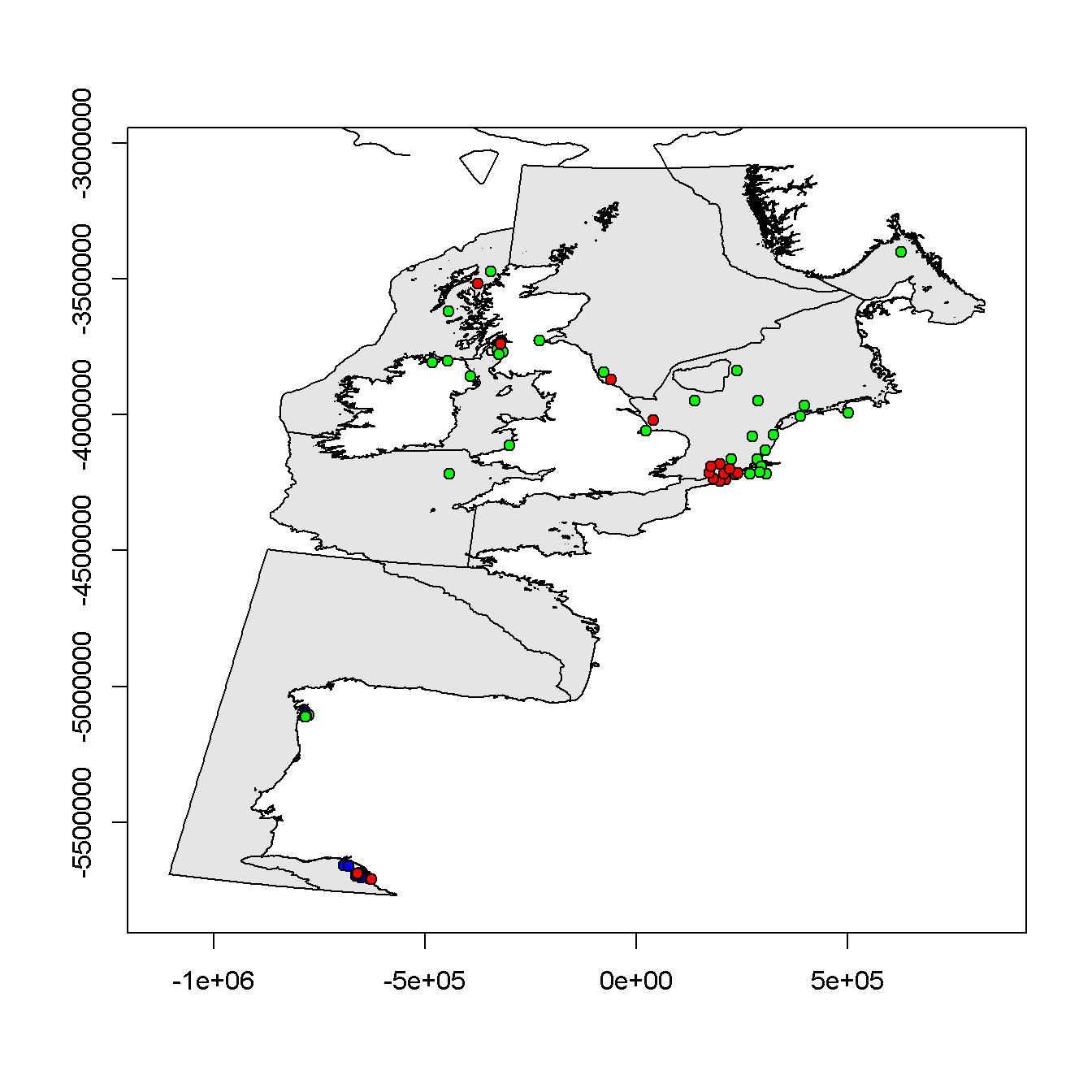##### BD100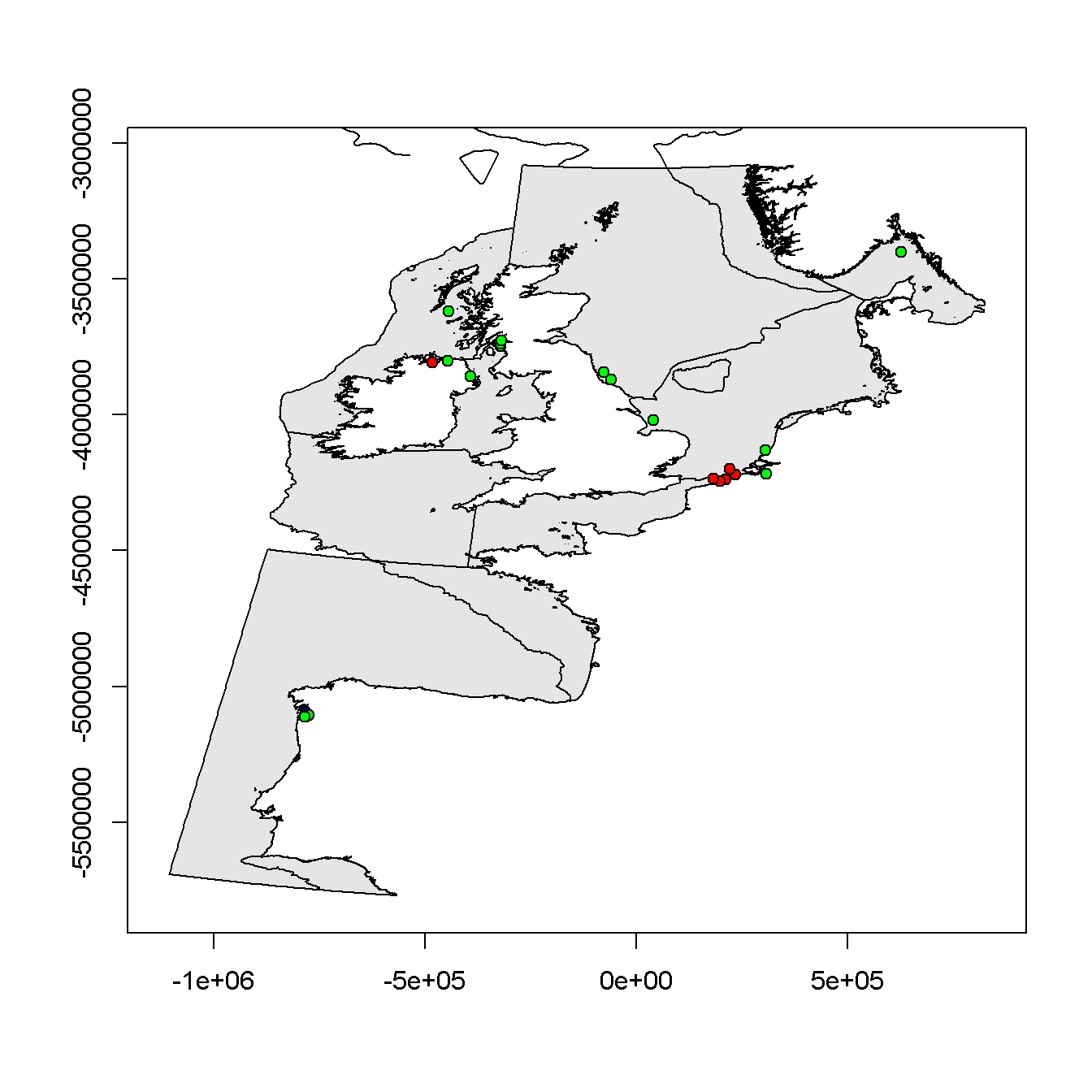##### BD153